# Introduction:

This tutorial will go over steps to parse through two different data sets and analyze their correlation. In this particular tutorial the two data sets used are temperature and energy usage data. The tempurature dataset measures temperature anomalies in the United States from the 1950-1981 average for each year and month from 1768 to 2013. The energy data set lists energy usage in the United States by industry over time from 1973-2012. In doing so, we are hoping to gain key insight into our environment, how energy usage relates to temperature, and global warming. Perhaps, our work today can explain key relationships that contribute to global warming. If we can identify such trends, then we can present insight on how to solve the global warming problem.

Throughout the tutorial, various data science steps will be explained. These steps can be categorized into 5 groups: Data Collection, Data Processing, Data Analysis and Visualization, Machine Learning and Hypothesis Testing, and Insight and Policy. Through following these steps you will be able to make conclusions from the data, in order to better understand the realtionship between temperature and energy usage.

### Tutorial Content:

The following sections will be covered in this tutorial:

# Installing Libraries:

The first step is to install the libraries that are imported below. To do this we used a package manager called anaconda. On Linux you can install anaconda by using this command:
 bash Anaconda3-4.3.1-Linux-x86_64.sh 

Once anaconda is installed you can then install the libraires used in this project. The main libraries used are Pandas, Numpy, matplotlib, scipy, and sklearn. The commands below will install these libraires:

conda install -c conda-forge matplotlib=2.0.2
conda install -c conda-forge scikit-learn=0.18.1
conda install -c conda-forge pandas=0.20.1
conda install -c conda-forge scipy=0.19.0
conda install -c conda-forge numpy=1.12.1


The next step is to import the proper classes and methods. This is done below.
In :
import pandas as pd
import numpy as np
from sklearn import linear_model as lm
import matplotlib.pyplot as plt
from scipy import stats
from sklearn.model_selection import train_test_split
from sklearn.metrics import mean_squared_error


In :
#Read energy data in from excel spreadsheet


Out:
Month Primary Energy Consumed by the Residential Sector Total Energy Consumed by the Residential Sector Primary Energy Consumed by the Commercial Sector Total Energy Consumed by the Commercial Sector Primary Energy Consumed by the Industrial Sector Total Energy Consumed by the Industrial Sector Primary Energy Consumed by the Transportation Sector Total Energy Consumed by the Transportation Sector Primary Energy Consumed by the Electric Power Sector Energy Consumption Balancing Item Primary Energy Consumption Total
0 1973-01-01 1313.816 1932.187 656.607 1079.310 2054.675 2701.524 1506.380 1509.552 1691.096 3.693 7226.265
1 1973-02-01 1150.011 1687.255 621.766 1001.920 1869.459 2460.752 1439.361 1442.127 1511.458 2.654 6594.709
2 1973-03-01 970.362 1497.067 490.154 884.437 1944.295 2579.613 1561.162 1564.016 1559.159 -0.934 6524.198
3 1973-04-01 709.631 1177.661 362.003 735.461 1924.207 2550.210 1479.971 1482.632 1470.152 -1.627 5944.336
4 1973-05-01 544.596 1015.008 289.109 696.114 2070.447 2741.810 1621.585 1624.436 1551.631 -2.817 6074.551
Congrats, you have succesfully read in the energy data contained in an excel spreadsheet! Easy enough, right? Now, if we take a look at our dataframe, we will see the column names are quite long. We simplify these column names below, then print the first five rows with Dataframe.head(5)
In :
#Change the column names, so they are shorter
energy_data.columns = ["Month", "P.E. Residential", "T.E. Residential", "P.E. Commerical", "T.E. Commercial",
"P.E. Industrial", "T.E. Industrial", "P.E. Transportation", "T.E. Transportation",
"P.E. Electric", "Energy Consumption Balancing Item", "P.E. Total Consumption"]

Out:
Month P.E. Residential T.E. Residential P.E. Commerical T.E. Commercial P.E. Industrial T.E. Industrial P.E. Transportation T.E. Transportation P.E. Electric Energy Consumption Balancing Item P.E. Total Consumption
0 1973-01-01 1313.816 1932.187 656.607 1079.310 2054.675 2701.524 1506.380 1509.552 1691.096 3.693 7226.265
1 1973-02-01 1150.011 1687.255 621.766 1001.920 1869.459 2460.752 1439.361 1442.127 1511.458 2.654 6594.709
2 1973-03-01 970.362 1497.067 490.154 884.437 1944.295 2579.613 1561.162 1564.016 1559.159 -0.934 6524.198
3 1973-04-01 709.631 1177.661 362.003 735.461 1924.207 2550.210 1479.971 1482.632 1470.152 -1.627 5944.336
4 1973-05-01 544.596 1015.008 289.109 696.114 2070.447 2741.810 1621.585 1624.436 1551.631 -2.817 6074.551
Now, we repeat the same process for our temperature dataset. This dataset is a text file. Therefore, the numpy method Numpy.genfromtxt() is used to load the data. This method returns an ndarray, containing the data in our textfile. In order to turn it into a dataframe, we can then pass our ndarray object into the Pandas.DataFrame constructor to make our temperature DataFrame.
In :
#loads the climate data

#dataframe representing the climate data
climate_data = pd.DataFrame(climateTable, columns=['Year', 'Month', 'Monthly Anomaly', 'Monthly Uncertainty', 'Annual Anomaly', 'Annual Uncertainty', 'Five Year Anomaly', 'Five Year Uncertainty', 'Ten Year Anomaly', 'Ten Year Uncertainty', 'Twenty Year Anomaly', 'Twenty Year Uncertainty'])

Out:
Year Month Monthly Anomaly Monthly Uncertainty Annual Anomaly Annual Uncertainty Five Year Anomaly Five Year Uncertainty Ten Year Anomaly Ten Year Uncertainty Twenty Year Anomaly Twenty Year Uncertainty
0 1768.0 9.0 -0.850 2.880 NaN NaN NaN NaN NaN NaN NaN NaN
1 1768.0 10.0 -1.578 3.386 NaN NaN NaN NaN NaN NaN NaN NaN
2 1768.0 11.0 -1.199 3.783 -0.471 2.380 NaN NaN NaN NaN NaN NaN
3 1768.0 12.0 -0.522 4.979 -0.204 2.390 NaN NaN NaN NaN NaN NaN
4 1769.0 1.0 0.118 4.856 -0.088 2.325 NaN NaN NaN NaN NaN NaN

# Data Processing:

The next step is to process the data. In this case we are going to merge our two dataframes (temperature and energy) into one data frame for easier access and comparison of data.

If you take a look back at the two dataframes below, you will see that date is used to identify unique rows in each dataframe. More specifically, you will see these rows overlap. We are going to try to use these dates to merge our dataframe. Unfortunately, while one can see these dates overlap, they are not formatted the same in each dataframe. As a result, the computer won't be able to recognize this intersection. So, in order to merge our dataframes, we must first format the dates in a way that is consistent across both dataframes. The easiest way to do this is to make use of Python's datetime object.Our energy data luckily already makes use of datetime objects, so all we have to do is process our temperature data. In order to do this, we first insert a "day" column to our temperature dataframe, initialized to all one's so it matches the energy dataset. Then, we take the year, month, and day columns and copy them into a new dataframe. We then call Pandas.to_datetime() to convert these fields into datetime objects. Lastly, we insert a new column, called "Date" that contains these newly formatted date objects, then drop the old "Year", "Month", and "Date" columns.
In :
#creates a new column in the format of year-month-day
climate_data['Day'] = np.ones(len(climate_data))
date = climate_data[['Year', 'Month', 'Day']].copy()
date = pd.to_datetime(date)
climate_data['Date'] = date

#drops the old date columns
climate_data = climate_data.drop('Year', axis=1)
climate_data = climate_data.drop('Month', axis=1)
climate_data = climate_data.drop('Day', axis=1)

#rearranges the columns
climate_data = climate_data[['Date', 'Monthly Anomaly', 'Monthly Uncertainty', 'Annual Anomaly',
'Annual Uncertainty', 'Five Year Anomaly', 'Five Year Uncertainty',
'Ten Year Anomaly', 'Ten Year Uncertainty', 'Twenty Year Anomaly',
'Twenty Year Uncertainty']]


Out:
Date Monthly Anomaly Monthly Uncertainty Annual Anomaly Annual Uncertainty Five Year Anomaly Five Year Uncertainty Ten Year Anomaly Ten Year Uncertainty Twenty Year Anomaly Twenty Year Uncertainty
0 1768-09-01 -0.850 2.880 NaN NaN NaN NaN NaN NaN NaN NaN
1 1768-10-01 -1.578 3.386 NaN NaN NaN NaN NaN NaN NaN NaN
2 1768-11-01 -1.199 3.783 -0.471 2.380 NaN NaN NaN NaN NaN NaN
3 1768-12-01 -0.522 4.979 -0.204 2.390 NaN NaN NaN NaN NaN NaN
4 1769-01-01 0.118 4.856 -0.088 2.325 NaN NaN NaN NaN NaN NaN
Now that the energy dataframe and climate dataframe have similar date columns, we can merge the two. However, the way in which we choose to merge is key. We perform an inner merge on our data; an inner merge takes the intersection of each dataframe, based on the specified keys. Once we have merged our two dataframes into one, we change the row indexing to represent dates. We change the index in our dataframe, because it allows us to easily look up data, based on date. The resulting dataframe is stored in climate_energy_data variable. The first five rows of climate_energy_data are printed below.
In :
#merges the two dataframes on the date
climate_energy_data = pd.merge(energy_data, climate_data, left_on = 'Month', right_on = 'Date')

#Reindex the dataset, so indices are Datetime objects
climate_energy_data = climate_energy_data.set_index(["Month"])
climate_energy_data = climate_energy_data.drop('Date', axis=1)
climate_energy_data.index.name = None


Out:
P.E. Residential T.E. Residential P.E. Commerical T.E. Commercial P.E. Industrial T.E. Industrial P.E. Transportation T.E. Transportation P.E. Electric Energy Consumption Balancing Item ... Monthly Anomaly Monthly Uncertainty Annual Anomaly Annual Uncertainty Five Year Anomaly Five Year Uncertainty Ten Year Anomaly Ten Year Uncertainty Twenty Year Anomaly Twenty Year Uncertainty
1973-01-01 1313.816 1932.187 656.607 1079.310 2054.675 2701.524 1506.380 1509.552 1691.096 3.693 ... -0.470 0.297 -0.266 0.054 -0.177 0.047 -0.101 0.024 -0.017 0.025
1973-02-01 1150.011 1687.255 621.766 1001.920 1869.459 2460.752 1439.361 1442.127 1511.458 2.654 ... 0.130 0.246 -0.263 0.046 -0.189 0.041 -0.114 0.024 -0.012 0.024
1973-03-01 970.362 1497.067 490.154 884.437 1944.295 2579.613 1561.162 1564.016 1559.159 -0.934 ... 1.555 0.279 -0.244 0.049 -0.198 0.038 -0.123 0.025 -0.013 0.024
1973-04-01 709.631 1177.661 362.003 735.461 1924.207 2550.210 1479.971 1482.632 1470.152 -1.627 ... -0.842 0.178 -0.124 0.046 -0.172 0.036 -0.115 0.026 -0.021 0.024
1973-05-01 544.596 1015.008 289.109 696.114 2070.447 2741.810 1621.585 1624.436 1551.631 -2.817 ... -0.408 0.313 -0.012 0.050 -0.181 0.035 -0.108 0.026 -0.027 0.023

5 rows × 21 columns

# Data Analysis and Visualization:

Our next step is to visualize the energy and temperature data. Often times, it is easier for us to gain insight into our dataset, or the problems we are trying to solve, by visualizing them. For example, a simple graph can give insights into trends and clusters within the data. This insight is extremely useful for analysis. We are going to make several graphs, showing trends in our energy and temperature data. To do so, we will rely upon Python's matplotlib library.First we will make several plots showing the distribution of our energy dataset over time. But, before we do so, we define a method getAvgs that will make our job a little easier. This method takes in a dataframe, and a sector S, and calculates the average energy usage per year, over sector S. The returned result is a list of averages, over the relevant years 1973 - 2013.
In :
#Gets the monthly average in Energy Usage for a sector over time.
#If no sector is specified, it computes monthly averages for total energy consumption (across all sectors)
def getAvgs(df, sector = None):
if( sector == None):
sector = "P.E. Total Consumption"
elif( sector == "Electric"):
sector = "P.E. Electric"
else:
sector = "T.E. " + sector

avgs = np.zeros( 2013 - 1973 + 1)
for i in range(1973, 2014):
temp = df[str(i)]

sum = np.sum(temp[sector].values)
avgs[i-1973] = sum / float(len(temp))

return avgs

Now, we define a method graph to make it easier to graph our data, and avoid reusing code. Our method takes in a list of X coordinates, Y coordinates, X-axis and Y-axis labels, and the type of plot to make (options are \"Line\" or \"Scatter\" plots. It then produces the specified type of plot, with a line of best fit.
In :
#Graphs X and Y datapoints, and sets the title, xLabel, and yLabel
#t is the type of graph.  Currently, Line Graphs and Scatter Plots have been implemented.
def graph(X, Y, title, xLabel, yLabel, t="Line"):
if( t == "Line"):
plt.plot(X, Y, label = "Data Points")
elif( t == "Scatter"):
plt.scatter(X, Y, label="Data Points")

plt.plot(X, np.poly1d(np.polyfit(X, Y, 1))(np.unique(X)), label="Best Fit Line")
slope, intercept = np.polyfit(X, Y, 1)
plt.legend(loc=0)
plt.title(title)
plt.xlabel(xLabel)
plt.ylabel(yLabel)
plt.show()
print("Slope of the line of best fit is: %f" %slope)


Using our methods above, we can easily compute and graph the yearly average in total monthly energy usage.

In :
#Plot average monthly energy usage per year over time
avgs = getAvgs(climate_energy_data)
years = np.arange(1973, 2014)

graph(years, avgs, "Average Monthly Energy Usage Across Sectors Over Time", "Year", "Energy Usage (Trillion BTU's)")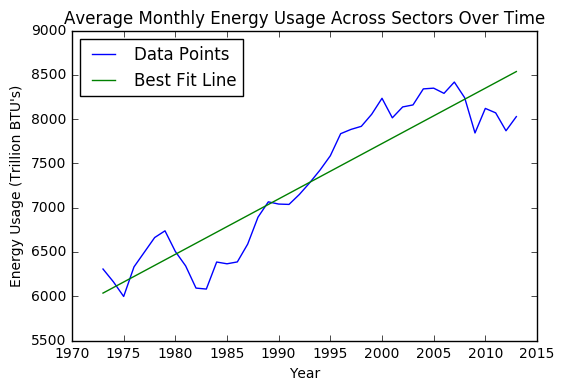Slope of the line of best fit is: 62.529150

Above we plotted the total average energy usage across all industries. In analyzing this graph and line of best fit it is clear that there is a direct relationship between energy usage and time. This means that as society has evolved the United States of America, energy usage has consistently increased.

Next we will create similar graphs for each industry, by computing the yearly averge in monthly energy usage for each industry.
In :
#Compute monthly averages for each individual sector
sectors = ["Residential", "Commercial", "Industrial", "Transportation", "Electric"]

for s in sectors:
avgs = getAvgs(climate_energy_data, s)
graph(years, avgs, "Average Monthly Energy Usage in the " + s + " Sector Over Time", "Year",
"Energy Usage (Trillion BTU's)")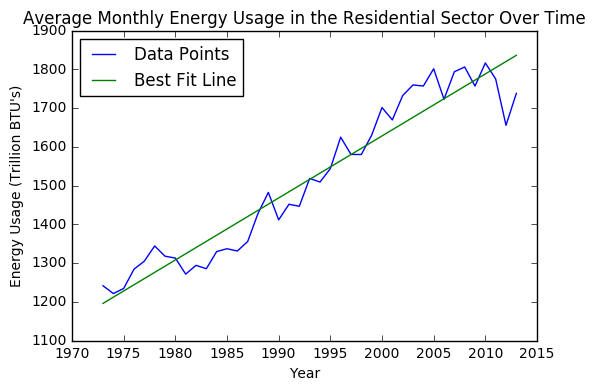Slope of the line of best fit is: 16.002637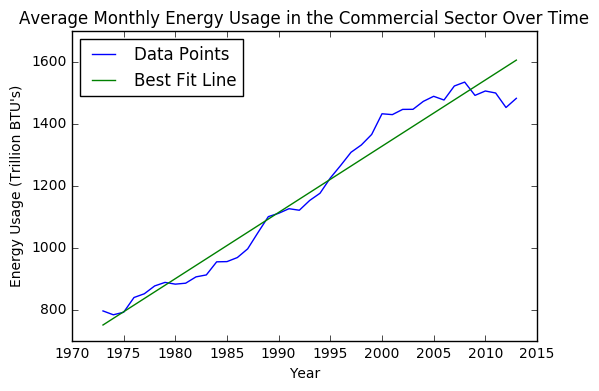Slope of the line of best fit is: 21.360539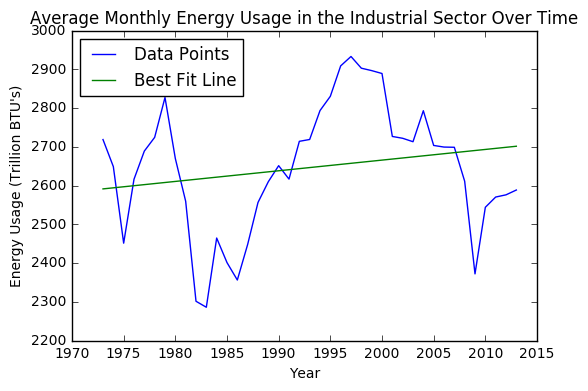Slope of the line of best fit is: 2.751618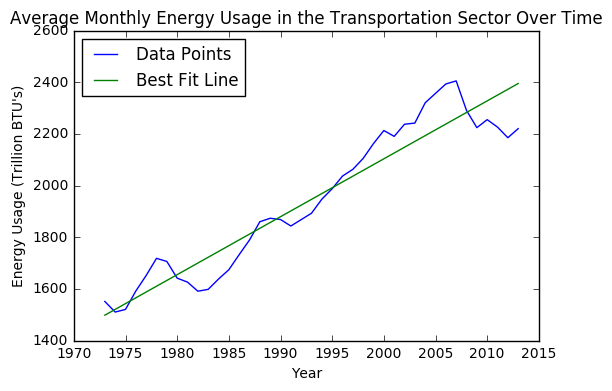Slope of the line of best fit is: 22.419588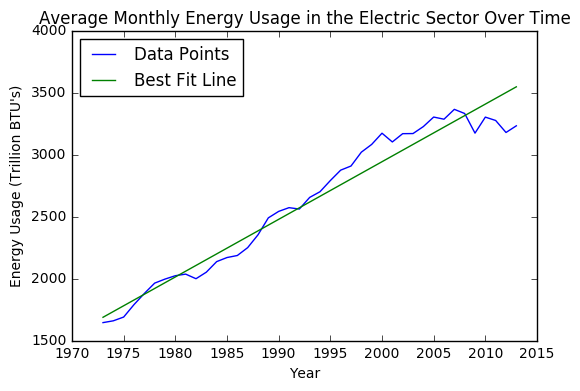Slope of the line of best fit is: 46.465518

As you can see from these graphs, most industries show a direct correlation between energy usage and time. With the exception of the industrial industry, we can clearly see energy increasing along with time.

Now, we are going to move on to our temperature data. We are going to use our original temperature dataframe, instead of our merged climate_energy_data dataframe. The reason we do this is because we are going to make two different plots - one over our merged domain (1973-2013) and another plot over our entire temperature domain.
To get started, we first do a quick indexing trick on our climate_data dataframe. We change the index so it is a datetime, similar to before, in order to allow us to easily access data based on temporal characteristics.

Next, we define a method called getAnomalies that functions similar to our getAvgs function. Next, we get the specific annual temperature anomalies, for each year in our defined range. Lastly, we return a list of our anomalies and all the years for which we have aggregated data.
In :
climate_data = climate_data.set_index(['Date'])
climate_data.index.name = None

In :
def getAnomalies(start, end):
end = end + 1
anomalies = np.zeros(end - start)
years = np.arange(start, end)

for y in range(start, end):
d = str(y) + '-06'
anomalies[y - start] = climate_data[d]['Annual Anomaly']
return anomalies, years


Great job! Now, we will make use of our getAnomalies method. We pass in 1973 and 2012, as start and end parameters respectively, to tell our method to get all anomalies during this time period. We then plot the returned lists by using our previously defined graph function. Both the code to produce this and the resulting plot can be seen below.

In :
anomalies, years = getAnomalies(1973, 2012)

graph(years, anomalies, "Change in Temperature Over Time From 1973 - 2012", "Years",
"Temperature Deviation from 1950-1970 Average (Degrees Celsius)")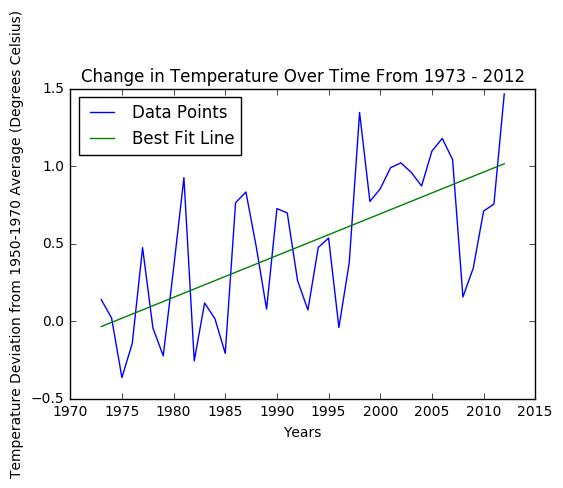Slope of the line of best fit is: 0.026938


As you can see from the plot above, there is a similar positive trend between temperature and time. As time increases, so does temperature. However, because of the variance of the plot, one might reasonably question the strength of this relationship. With temperature anomaly constantly jumping up and down, can we definitively say that time is causing temperature to increase?

The answer to this question becomes more apparent below, when we expand our effective domain. If we extend our time period farther back, to 1820, we can more clearly see a stronger relationship between time and temperature.

In :
anomalies, years = getAnomalies(1820, 2012)

graph(years, anomalies, "Change in Temperature Over Time From 19820 - 2012", "Years",
"Temperature Deviation from 1951-1980 Average (Degrees Celsius)")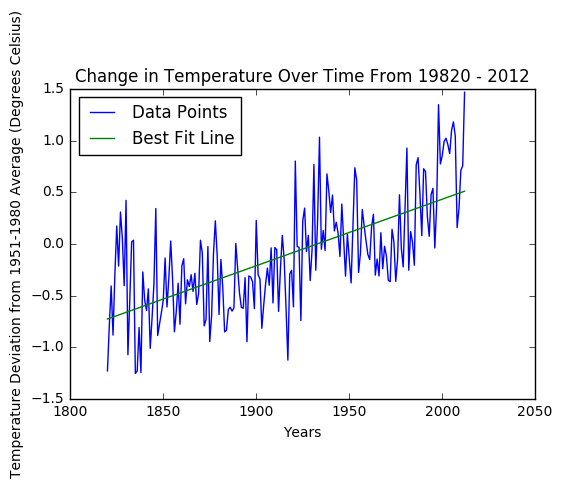Slope of the line of best fit is: 0.006442


Now, we can see a nice trend upwards between temperature anomaly and time. As a result, we can now reasonably conclude that: as date increases, so does temperature.

# Hypothesis Testing and Machine Learning

Now, we are going to delve deeper into the hypothesis testing and machine learning aspects of data science. Through data visualization and analysis, we were able to show that temperature and energy usage both increase with time. From this, we would now like to hypothesize that temperature and energy usage are directly correlated (i.e. an increase in one leads to an increase in the other). Being able to prove such a hypothesis would give key insight into global warming and how to combat it.

We are going to start by taking our hypothesis and calculating the pearson correlation coefficient for our temperature and energy data. If you are unfamiliar with what this coefficient is, or what it means, then check out this Wikipedia page. Luckily for us, there is a well defined stats library in scipy that can help us calculate this in one line:

In :
stats.pearsonr(climate_energy_data['P.E. Total Consumption'], climate_energy_data['Monthly Anomaly'])

Out:
(0.30987579239266005, 2.4159757806075858e-12)

The result of the code above is a tuple of two values. The first value is the pearson's correlation coefficient. A correlation coefficient of +1 or -1 is indicative of a perfect linear relationship. With a correlation coefficient of roughly .3099, we can conclude that there is a weak positive correlation between our two datasets. As a result, we cannot definitively claim that temperature and energy are directly related.

The second value is our two-tailed p-value. This indicates the probability that an uncorrelatd system produced similar results. As we can see, our two-tailed p-value is miniscule and basically zero. So, we can safely conclude that an uncorrelated system would not produce similar results.

In essence, we have just shown that there is a weak correlation between our energy and temperature data. In other words, there is some correlation between our two datasets, but not enough for us to definitively say there is a linear relationship. This makes sense, too. To visualize and make more sense of this weak correlation, we will take a moment to graph Annual Temperature Anomaly vs. Energy Consumption. The code below uses our getAvgs and getAnomalies to get the correct data, then produces a plot.

In :
avgs = getAvgs(climate_energy_data)
avgs = avgs[1:41]
anomalies, years = getAnomalies(1973, 2012)

graph(avgs, anomalies, "Anomalies and Energy Consumption", "Energy Consumption",
"Yearly Temp Anomalies")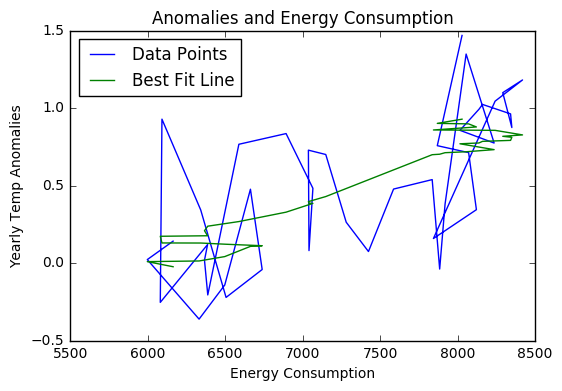Slope of the line of best fit is: 0.000393


Looking at this plot, it makes sense that there is a weak correlation. The plot above looks like madness, which would cause one to doubt our initial claim that temperature and energy are correlated. However, in the Data Visualization section, we proved that both temperature anomlay and energy consumption increase with time. As a result, it makes sense that we cannot definitively prove our data is uncorrelated either.

Now, we are going to transition away from hypothesis testing, and focus on machine learning. The whole concept of machine learning is being able to generalize, or learn, a predictive model based on some sort of training data. With any luck, this model will be able to make accurate predictions on a separate dataset, used for testing.

To develop a predictive model, we are going to use scikit-learn, a powerful Python library that handles much of the behind-the-scenes work for us. Scikit-learn makes ML fairly easy, as it reduces numerous lines of complex code down to one function call. To start off, we are going to define two functions.

First, we are going to define a function trainTestSplit that takes our dataset and splits it into training and test data. This is necessary, because we want to train our predictive model on our training data and evaluate its accuracy using test data. Imagine you are taking an exam, and the test questions and answers are leaked to you. Obviously, exam scores will be high simply because students had access to the questions and answers, and as a result, the exam would not be an accurate evaluation of a student's ability to learn. The same idea can be applied to machine learning. If we peek at the answers (our test data) too early, we are cheating. As a result, it is important to maintain this train/test split. In order to implement the actual splitting of our data, we rely on scikit-learn to reduce numerous lines of code to one method call.

Our second method, linear_model, trains a basic linear regression model using scikit-learn. In linear regression, our goal is to learn a vector of weights, w, for which we can compute a prediction. Say we have an example, X, represented by a vector. By computing the dot product of w and X, we can make a prediction for example X. In essence, this simple concept is linear regression.

The code for both of these two methods is below. As you can see, scikit-learn makes implementing these methods extremely easy. If you are interested in more machine learning models, feel free to checkout the scikit-learn's online documentation. The library is extremely powerful for machine learning purposes, and can be a good place for a beginner to start.

In :
def trainTestSplit(X, Y):
X_train, X_test, Y_train, Y_test = train_test_split(X, Y, test_size=0.2, random_state=42)

return X_train, X_test, Y_train, Y_test

In :
def linear_model(X, Y):

#creates the linear regression model
reg = lm.LinearRegression()
reg.fit(X, Y)

return reg


Now, we can train our linear regression model. We are going to try to predict energy consumption and temperature anomaly based on date. We start off by dropping all rows containing empty values from our climate_energy_data dataframe. Then, we aggregate all our data by creating a 2d-array of examples, with each row formatted as [year, month, day] and a 1d-array of corresponding labels. We then call our trainTestSplit() method on our two arrays, to split our training from our test data. Lastly, we train a linear regresion model by feeding our training data into our linear_model() function.

In :
total_data = climate_energy_data.dropna()

X = np.c_[total_data.index.year, total_data.index.month, total_data.index.day]
Y = total_data['P.E. Total Consumption'].values

X_train, X_test, Y_train, Y_test = trainTestSplit(X, Y)

energy_reg = linear_model(X_train, Y_train)


We have just succesfully trained our linear regression model, and can now make predictions using it. We will start off by making predictions on our test set. Scikit-learn makes this extremely easy, we simply call scikit-learn's predict method, passing in the feature vectors from our test set. Scikit-learn then returns a 1d-array of predicted values, we call this array Yhat.

In :
Yhat = energy_reg.predict(X_test)


Now that we have made our predictions, we need a way to evaluate the performance of our learned model. The way we go about evaluating our accuracy, is to define our loss function. If you want to read up on loss functions, visit this Wikipedia Page, but essentially they are a way of penalizing wrong predictions.

For our model, we are going to use squared loss. The formula to compute our loss function is: SUM((Y-Yhat)2), where Y is the correct value and Yhat is our predicted value. Using this formula, we are going to define two functions. The first method, getLoss(), calculates and returns our loss, given true values and predicted values. The second method, plotLoss(), makes a scatter plot of our loss, and draws a horizontal line, indicating our average loss for our test set.

In :
def getLoss(Ytrue, Yhat):
return (Yhat - Ytrue)**2

In :
def plotLoss(loss, avg_loss):
N = len(loss)
X = np.arange(N) + 1

plt.scatter(X, loss)
plt.plot(X, np.ones(N) * avg_loss, label="Average Loss", c="red", linewidth=2.0)
plt.title("Squared Loss")
plt.ylabel("Loss value")
plt.legend(loc=0)
plt.show()


Next, we can use the two methods we just defined to calculate our loss. We use scikit-learn's mean_squared_error method to calculate our average squared loss. Then, we plot loss and average loss and print out the values for total and average loss, to more easily visualize these values. We will see that the loss for our predictions is extremely high, but do not worry. Our model is performing fine, rather the energy dataset uses extremely large values, so this is expected.

In :
loss = getLoss(Y_test, Yhat)
totalLoss = np.sum(loss)

avg_loss = mean_squared_error(Y_test, Yhat)

plotLoss(loss, avg_loss)
print("Total Loss: %s" % totalLoss)
print("Average Loss: %s" % avg_loss)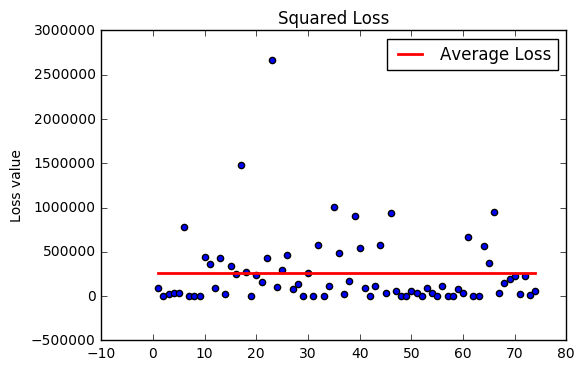Total Loss: 19099035.1904
Average Loss: 258095.07014


Now, we repeat the process for our temperature data. We want to develop a linear regression model to help us predict temperature anomaly, given a date.

Remember to use the same process:

1. Split train/test data.
2. Train model on trainig data, using our linear_model() method.
3. Make our predictions on our test set.
4. Compute and plot loss
In :
Y = total_data['Monthly Anomaly'].values
X_test, X_train, Y_test, Y_train = trainTestSplit(X, Y)

temp_reg = linear_model(X_train, Y_train)

In :
Yhat = temp_reg.predict(X_test)

In :
loss = getLoss(Y_test, Yhat)
totalLoss = np.sum(loss)

avg_loss = mean_squared_error(Y_test, Yhat)

print("Total Loss: %s" % totalLoss)
print("Average Loss: %s" % avg_loss)
plotLoss(loss, avg_loss)

Total Loss: 268.964826292
Average Loss: 0.911745173873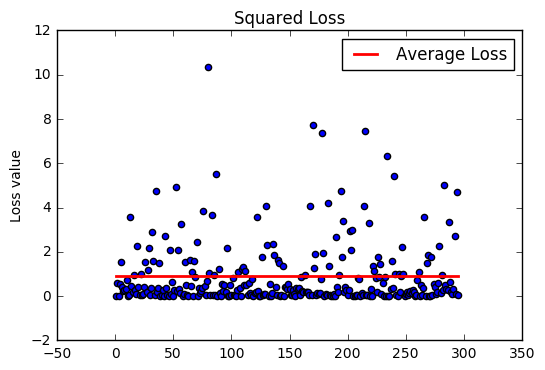Now, let's try to predict the future. For example, what will temperature and energy consumption look like in January 2050? You may not know the answer to this off the top of your head, but we can use our learned model to give us an idea of what to expect. Unlike before, we will not know the actual values of these predictions, as we are predicting the future, but we know our learned models perform decently well.

Let's try making predictions for a few dates: August 1st, 2020, November 8th, 2024, May 10th, 2030, May 18th, 2032, and February 24th 2035. We start off by defining an nd-arrary of the feature vectors we would like to predict. This ndarray contains the dates previously listed, with each row being of the format [Year, Month, Day].

In :
X = np.array([[2020, 8, 1],
[2024, 11, 8],
[2032, 5, 10],
[2035, 2, 24]])


Then, we make predictions on using this nd-array

In :
Yhat_energy = energy_reg.predict(X)
Yhat_temp = temp_reg.predict(X)

print("Energy Predictions: %s" % str(Yhat_energy))
print("Temperature Predictions: %s" % str(Yhat_temp))

Energy Predictions: [  9396.5706769    9623.36301856  10384.30589298  10688.86935783]
Temperature Predictions: [ 1.52348165  1.70713331  2.00791078  2.11654447]


As we can see, these numbers continue increasing with time. On February 24th, 2035, we can expect energy usage to total 10,688.87 Trillion BTU's and energy anomaly to be 2.11 degrees higher than the 1951 - 1980 average. Given the state of our planet, and our desperate need to reduce our carbon footprint, wouldn't it make sense to reduce our energy usage? Not necessarily. The answer to this question, and the reason why is further explained in the next section, Insight and Policy

# Insight and Policy:

In this tutorial, we attempted to show a positive correlation between energy usage and temperature. When we were unable to, we turned our efforts to developing a predictive model, that used historical trends to predict future energy usage and temperature.

In our attempt to gain insight into global warming, we were unable to confirm the correlation between energy usage and temperature. However, that does not mean they are uncorrelated. Rather, it means we did not do enough work today to prove the existence of such a correlation. Perhaps, we can show this by gathering new data from www.data.gov, or performing more significant data processing. Through further exploration, we may be able to confirm or reject this hypothesis, but for now, the question remains unanswered.

Furthermore, we set out on a quest to see how energy usage and temperature change over time. From our visualizations, analysis, and machine learning, we can confidently say that energy usage and temperature will increase in the future. However, that does not necessarily mean our global warming problem is getting worse. Many nations worldwide are investing heavily in renewable sources of energy. As a result, energy usage will still increase, but will have a less significant impact on global warming and rising temperatures. Yet, despite this push for renewable energy, temperatures continue to rise. One logical answer to this, is that society is still very dependent on fossil fuels making the transition to clean energy a lengthy process.